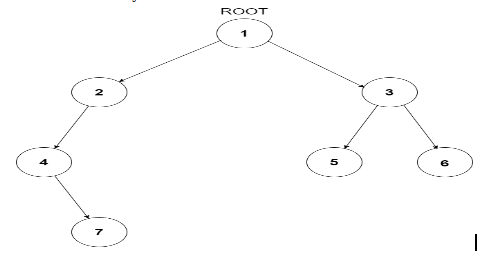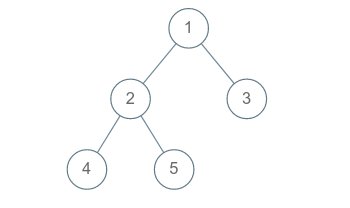Problem title
Difficulty
Avg time to solve

Chocolate Bar
Hard
45 mins
Rearrange The Array
Easy
--
Free from cubes
Moderate
15 mins
Fishmonger
Hard
30 mins
Three Factors
Moderate
25 mins
Left Right Node Balancing
Easy
15 mins
Longest Consecutive Sequence In A Binary Tree
Moderate
15 mins
Maximum Difference Between Node And Ancestor
Easy
10 mins
Count Distinct Elements In Every Window
Moderate
15 mins
Convert A Binary Tree To A Circular Doubly Linked List
Hard
10 mins3

# Kth ancestor of a node in binary tree

Difficulty: HARD
Avg. time to solve
50 min
Success Rate
35%

Problem Statement

#### The Kth ancestor of a node in a binary tree is the Kth node in the path going up from the given node to the root of the tree. Refer to sample test cases for further explanation.

##### Note:
``````1. The given node 'TARGET_NODE_VAL' is always present in the tree.
2. The value of each node in the tree is unique.
3. Notice that the Kth ancestor node if present will always be unique.
``````
##### Input Format:
``````The first line contains a single integer T representing the number of test cases.

The first line of each test case will contain the values of the nodes of the tree in the level order form ( -1 for NULL node) Refer to the example for further clarification.

The second line of each test case will contain two space-separated integers K and TARGET_NODE_VAL which are the value of the Kth ancestor to be found of the node and the value of the node given for which the Kth ancestor is to be computed respectively.

Example:
Consider the binary tree:
````````````The input of the tree depicted in the image above will be like:
1
2 3
4 -1 5 6
-1 7 -1 -1 -1 -1
-1 -1

Explanation :
Level 1 :
The root node of the tree is 1

Level 2 :
Left child of 1 = 2
Right child of 1 = 3

Level 3 :
Left child of 2 = 4
Right child of 2 = null (-1)
Left child of 3 = 5
Right child of 3 = 6

Level 4 :
Left child of 4 = null (-1)
Right child of 4 = 7
Left child of 5 = null (-1)
Right child of 5 = null (-1)
Left child of 6 = null (-1)
Right child of 6 = null (-1)

Level 5 :
Left child of 7 = null (-1)
Right child of 7 = null (-1)

The first not-null node (of the previous level) is treated as the parent of the first two nodes of the current level. The second not-null node (of the previous level) is treated as the parent node for the next two nodes of the current level and so on.

The input ends when all nodes at the last level are null (-1).
``````
##### Output Format:
``````For each test case, print the value of the Kth ancestor of the node in a separate line. If it doesn’t exist, then print -1.
``````
##### Note:
``````You do not need to print anything, it has already been taken care of. Just implement the given function.
``````
##### Constraints:
``````1 <= T <= 100
1 <= N <= 3000
1 <= K <= 10^9
1 <= NODE_VAL <= 10^9

Time Limit: 1 sec
``````
##### Sample Input 1:
``````1
1 2 3 4 5 -1 -1 -1 -1 -1 -1
5 2
``````
##### Sample Output 1:
``````1
``````
##### Explanation for sample input 1:``````The path from the given node 5 to root 1 is 5 -> 2 -> 1
So the 2nd ancestor of 5 is 1
``````
##### Sample Input 2:
``````1
1 2 3 4 5 -1 -1 -1 -1 -1 -1
4 1
``````
##### Sample Output 2:
``````2
``````
##### Explanation for sample input 2:``````The path from the given node 4 to root 1 is 4 -> 2 -> 1
So  the 1st ancestor of 4 is 2
``````Console